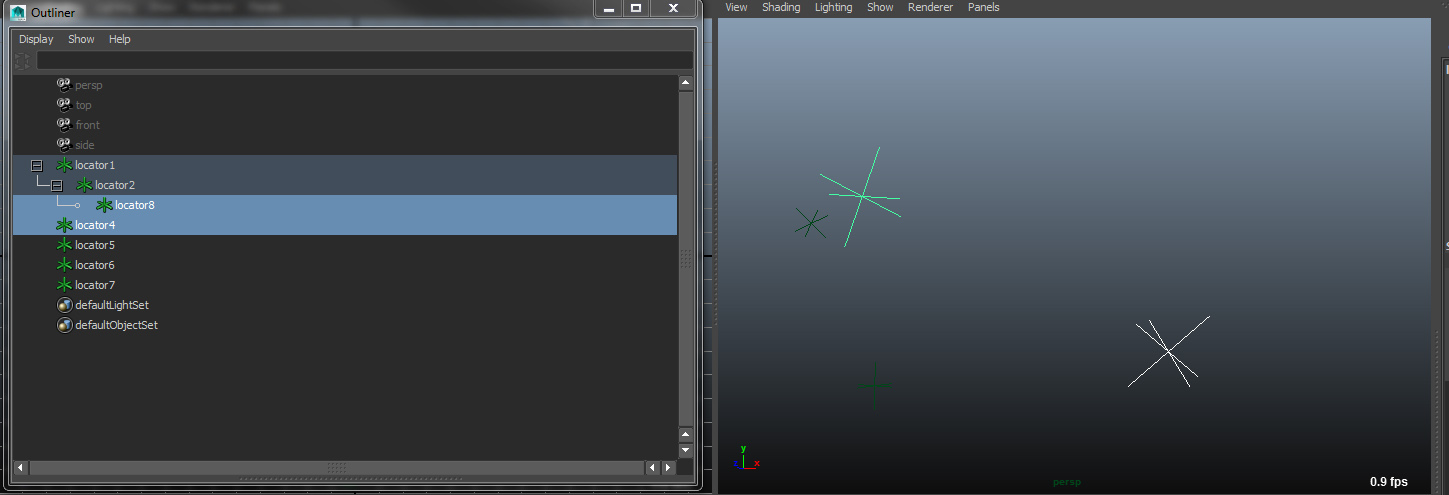# Match transfroms in Maya

Currently we are switching our workflows from Softimage to Maya. One small, but essential feature we miss, is the good old match all transforms functionality. This function matches the translation, rotation and transforms from one object to another.Here is my way to adapt our current workflow to Maya.

```import maya.cmds as cmd
import maya.mel

# matchtransforms ===========================================
# Matches the transfroms from one object to multiple another objects in worldspace
# @param inObjects - Current Maya Selection
# @param inOperation - Integer(0=All Transforms, 1=Translation, 2=Rotation, 3=Scaling)
# @return true.
def matchtransforms(inObjects, inOperation):
#Find the object from where to copy the transforms
oTo = inObjects[len(inObjects) - 1]
#Find all objects that should match the transforms of the "oTo" Object
oFrom = inObjects
oFrom.pop(len(inObjects) - 1)
#Match all transforms
if(inOperation == 0):
pivotTranslate = cmds.xform( oTo, q = True, m = True, ws = True ) #m=matrix,ro=rotation,t=transfrom
cmds.xform(oFrom, a=True, m=pivotTranslate)
#Match translation
if(inOperation == 1):
pivotTranslate = cmds.xform(oTo, q = True, t = True, ws = True ) #m=matrix,ro=rotation,t=transfrom
cmds.xform(inObjToMatch, a=True, t=pivotTranslate)
#Match rotation
if(inOperation == 2):
pivotTranslate = cmds.xform(oTo, q = True, ro = True, ws = True ) #m=matrix,ro=rotation,t=transfrom
cmds.xform(inObjToMatch, a=True, ro=pivotTranslate)
#Match scale
if(inOperation == 3):
pivotTranslate = cmds.xform(oTo, q = True, s = True, ws = True ) #m=matrix,ro=rotation,t=transfrom
cmds.xform(inObjToMatch, a=True, s=pivotTranslate)
return

#Get current Selecton
selection= cmds.ls(selection=True )
matchtransforms(selection,0)

```